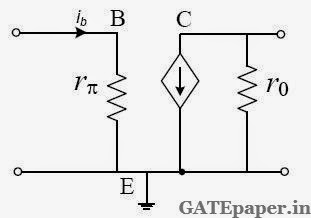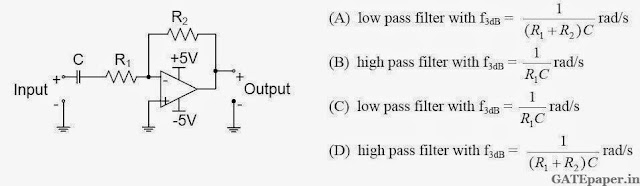### GATE 2012 ECE Video Solutions on Analog Circuits (Analog Electronics)

1. The current ib through the base of a silicon NPN transistor is 1+0.1cos (10000πt) mA. At 300oK, the rπ in the small signal model of the transistor is ________ (in Ohms).a. 250
b. 27.5
c. 25
d. 22.5

2. The impedance looking into nodes 1 and 2 in the given circuit is________ (in Ohms).a. 50
b. 100
c. 5K
d. 10.1 K

3. The circuit shown is a4. The voltage gain Av, of the circuit shown below isa. |Av| ≡ 200
b. |Av| ≡ 100
c. |Av| ≡ 20
d. |Av| ≡ 10